# Form a complete graph for each of the following. Include all intercepts and asymptotes - 3x+2 x +...

Rational graphs worksheet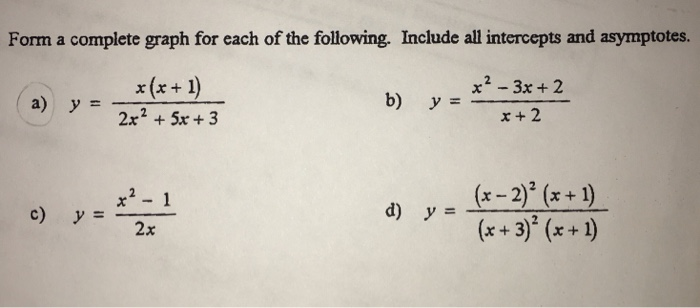Form a complete graph for each of the following. Include all intercepts and asymptotes - 3x+2 x +2 x(x+1 y= (x-2)2 (x +1) x3 (x +1 d) c) y2x y =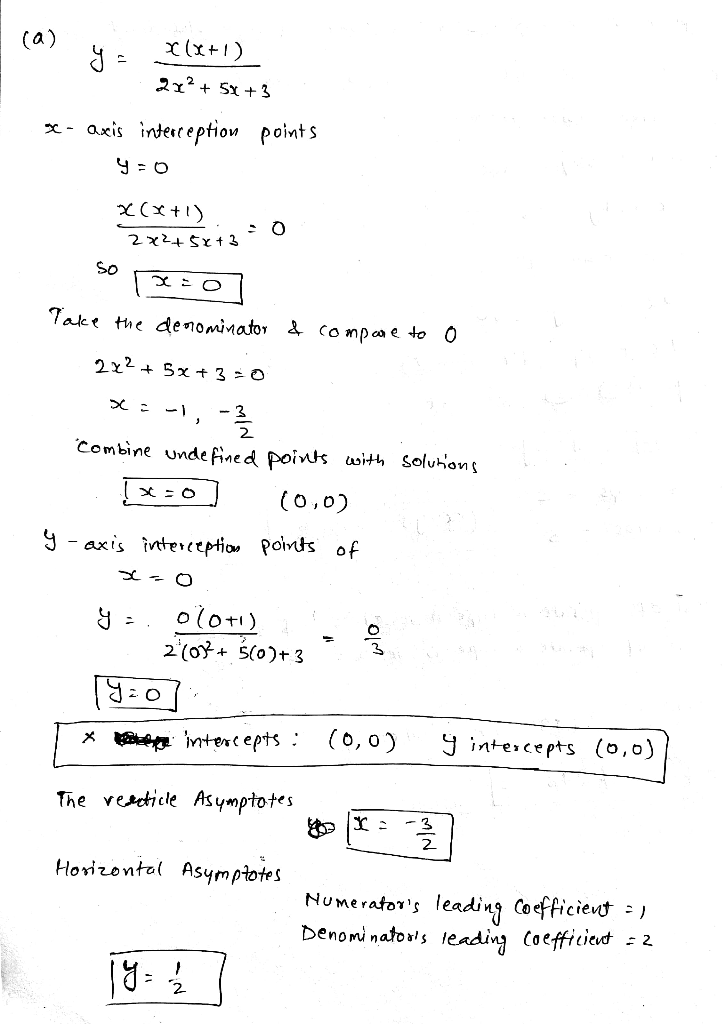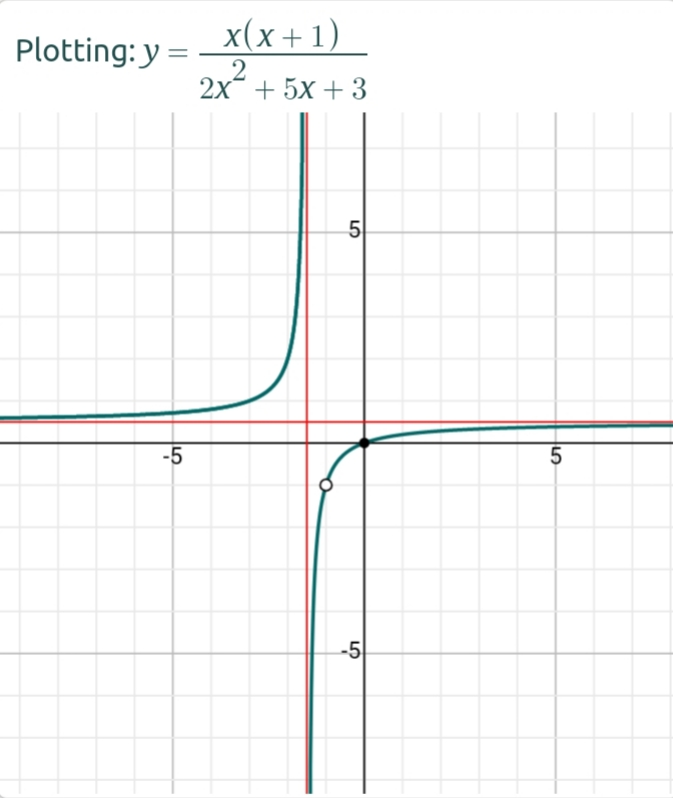##### Add Answer of: Form a complete graph for each of the following. Include all intercepts and asymptotes - 3x+2 x +...
Similar Homework Help Questions
• ### find all asymptotesfind all asymptotes, x-intercepts and y-intercepts for thegraph of each rational function and sketch the graph of thefunction.f(x)=

• ### the problem is as follows=== Identify all asymptotes, the y intercept, all x intercepts for the graph f(x)

the problem is as follows=== Identify all asymptotes, the y intercept, all x intercepts for the graph f(x). Then scetch the graph. f(X)= 2x^2-5x-3 --------- x^2-9 Thanks for your help

• ### (a)graph x^2/16 - y^2/25 = 1 show how you arrived at your graph by determining the (b)x-intercepts, (c)extent of the graph and the (d)asymptotes

(a)graph x^2/16 - y^2/25 = 1 show how you arrived at your graph by determining the (b)x-intercepts, (c)extent of the graph and the (d)asymptotes

• ### Please tell me which options I need to select and what I have to type in. Thank you! 3-3x For the given rational function f(x)- x- find the following (A) Find the intercepts for the graph. (B) Determi...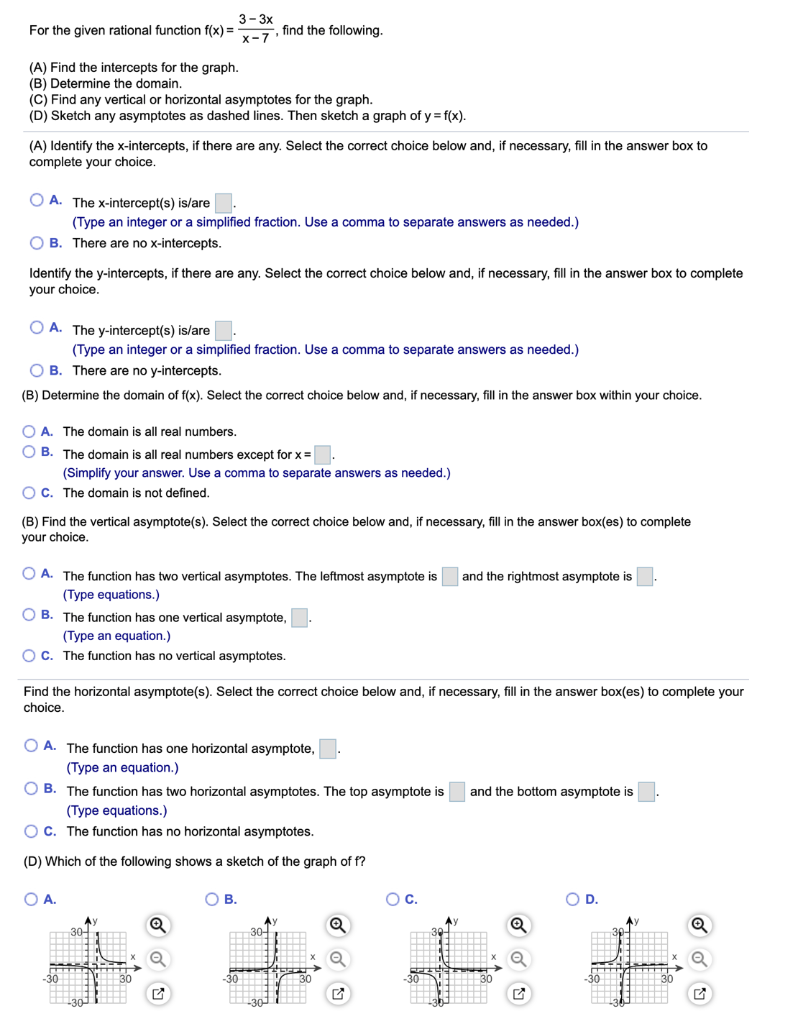Please tell me which options I need to select and what I have to type in. Thank you! 3-3x For the given rational function f(x)- x- find the following (A) Find the intercepts for the graph. (B) Determine the domain. (C) Find any vertical or horizontal asymptotes for the graph (D) Sketch any asymptotes as dashed lines. Then sketch a graph of y f(x) (A) Identify the x-intercepts, if there are any. Select the correct choice below and, if necessary,...

• ### 4 and 5 in the bottom pls help ge Algebra Final B y College of Denver SP 2019 4. A rational function fix) has asymptotes of x 2, x 2, and y- 3. The x and y intercepts are (-1, 0), (1, 0) and 0, 3)...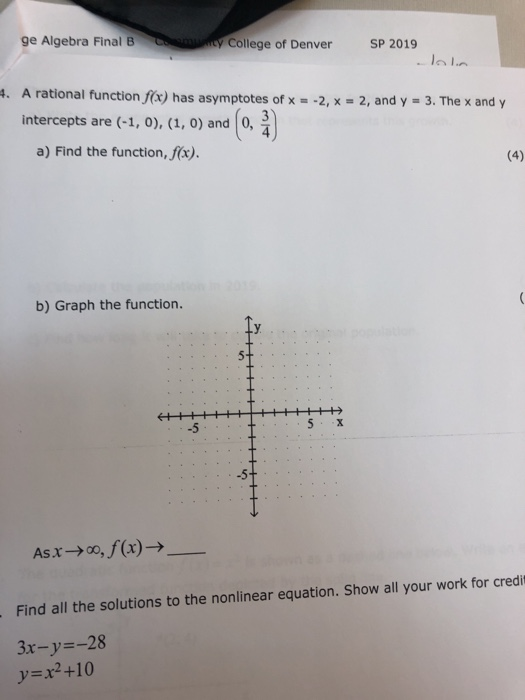4 and 5 in the bottom pls help ge Algebra Final B y College of Denver SP 2019 4. A rational function fix) has asymptotes of x 2, x 2, and y- 3. The x and y intercepts are (-1, 0), (1, 0) and 0, 3) a) Find the function, fax). b) Graph the function. -5 -5 Asx-oo, f(x)-> Find all the solutions to the nonlinear equation. Show all your work for credit 3x-y--28 У x2 +10 ge Algebra Final...

• ### x and y intercepts, and vertical, horizontal and slant asymptotes

g(x)= (x2-3x-4)/ (x-1)Find the x- intercepts and y- intercepts, vertical asymptotes, horizontal asymptotes, and slant asymptotes.

• ### asymptotes

Consider the rational functionf(x) = -2x+1/x^2 + 6x +9a. What’s the domain of f (x) ?b. What are the x and y intercepts (if any) of the graph of f (x) ?c. What are the vertical asymptotes of f (x) ? (if any)d. What are the horizontal asymptotes of f (x) ? (if any)e. Sketch the graph.

• ### (a) graph x squared/16 - y squared/25 = 1 show how you arrived at your graph by determining the (b) x-intercepts, (c) extent of the graph and the (d) asymptotes

(a) graph x squared/16 - y squared/25 = 1 show how you arrived at your graph by determining the (b) x-intercepts, (c) extent of the graph and the (d) asymptotes

• ### i need to find the domain, intercepts and any asymptotes of f(x)=(x^2+3X-4)/(x+1) but when i graph it it's a hyperbola and its throwing me off

i need to find the domain, intercepts and any asymptotes of f(x)=(x^2+3X-4)/(x+1) but when i graph it it's a hyperbola and its throwing me off!! please help!!

• ### asymptotes, graphs, holes, y intercepts, zeros, domain. range, function increasing and...

I have to graph thisy=f(x) = (x-1)/ x(x+1)I have to show how i GOT and label all axes, asymptotes, graphs, holes, y intercepts, zeros, domain. range, function increasing and decreasing, even, odd orneither.My graph doesnt seem to have a symptote..

Need Online Homework Help?